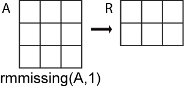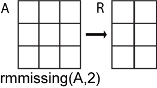# rmmissing

Remove missing entries

## Syntax

``R = rmmissing(A)``
``R = rmmissing(A,dim)``
``R = rmmissing(___,Name,Value)``
``````[R,TF] = rmmissing(___)``````

## Description

example

````R = rmmissing(A)` removes missing entries from an array or table. If `A` is a vector, then `rmmissing` removes any entry that contains missing data. If `A` is a matrix or table, then `rmmissing` removes any row that contains missing data.Missing values are defined according to the data type of `A`: `NaN` — `double`, `single`, `duration`, and `calendarDuration``NaT` — `datetime``<missing>` — `string``<undefined>` — `categorical``{''}` — `cell` of character vectors If `A` is a table, then the data type of each column defines the missing value for that column.```

example

````R = rmmissing(A,dim)` specifies the dimension of `A` to operate along. By default, `rmmissing` operates along the first dimension whose size does not equal 1.```

example

````R = rmmissing(___,Name,Value)` specifies additional parameters for removing missing entries using one or more name-value arguments. For example, you can use `rmmissing(A,'MinNumMissing',n)` to remove rows of `A` that contain at least `n` missing values.```

example

``````[R,TF] = rmmissing(___)``` also returns a logical vector corresponding to the rows or columns of `A` that were removed.```

## Examples

collapse all

Create a vector with `NaN` values and remove each `NaN`.

```A = [1 3 NaN 6 NaN]; R = rmmissing(A)```
```R = 1×3 1 3 6 ```

Remove incomplete rows from a table with multiple data types.

First, create a table whose variables include `categorical`, `double`, and `char` data types.

```A = table(categorical({''; 'F'; 'M'}),[45; 32; NaN],{''; 'CA'; 'MA'},[6051; 7234; NaN],... 'VariableNames',{'Gender' 'Age' 'State' 'ID'})```
```A=3×4 table Gender Age State ID ___________ ___ __________ ____ <undefined> 45 {0x0 char} 6051 F 32 {'CA' } 7234 M NaN {'MA' } NaN ```

Remove any row of the table that contains missing data.

`R = rmmissing(A)`
```R=1×4 table Gender Age State ID ______ ___ ______ ____ F 32 {'CA'} 7234 ```

Only remove rows with missing values in the `Age` or `ID` table variables.

`R = rmmissing(A,'DataVariables',{'Age','ID'})`
```R=2×4 table Gender Age State ID ___________ ___ __________ ____ <undefined> 45 {0x0 char} 6051 F 32 {'CA' } 7234 ```

Alternatively, use the `isnumeric` function to identify the numeric variables to operate on.

`R = rmmissing(A,'DataVariables',@isnumeric)`
```R=2×4 table Gender Age State ID ___________ ___ __________ ____ <undefined> 45 {0x0 char} 6051 F 32 {'CA' } 7234 ```

Create a matrix with missing data and remove any column (second dimension) containing two or more missing values. Return the new matrix and the logical row vector that indicates which columns of `A` were removed.

```A = [NaN NaN 5 3 NaN 5 7 NaN 9 2; 8 9 NaN 1 4 5 6 5 NaN 5; NaN 4 9 8 7 2 4 1 NaN 3]```
```A = 3×10 NaN NaN 5 3 NaN 5 7 NaN 9 2 8 9 NaN 1 4 5 6 5 NaN 5 NaN 4 9 8 7 2 4 1 NaN 3 ```
`[R,TF] = rmmissing(A,2,'MinNumMissing',2)`
```R = 3×8 NaN 5 3 NaN 5 7 NaN 2 9 NaN 1 4 5 6 5 5 4 9 8 7 2 4 1 3 ```
```TF = 1x10 logical array 1 0 0 0 0 0 0 0 1 0 ```

## Input Arguments

collapse all

Input data, specified as a vector, matrix, cell array of character vectors, table, or timetable.

• If `A` is a `timetable`, then `rmmissing(A)` removes any row of `A` containing missing data and also removes the corresponding time vector element. If the time vector contains a `NaT` or `NaN`, then `rmmissing(A)` removes it from the time vector and also removes the corresponding row of `A`.

• If `A` is a cell array or a table with cell array variables, then `ismissing` only detects missing elements when the cell array contains character vectors.

Dimension to operate along, specified as 1 or 2. By default, `rmmissing` operates along the first dimension whose size does not equal 1.

Consider an `m`-by-`n` input matrix array, `A`:

• `rmmissing(A,1)` removes rows of `A` that contain missing data.• `rmmissing(A,2)` removes columns of `A` that contain missing data.### Name-Value Arguments

Specify optional pairs of arguments as `Name1=Value1,...,NameN=ValueN`, where `Name` is the argument name and `Value` is the corresponding value. Name-value arguments must appear after other arguments, but the order of the pairs does not matter.

Before R2021a, use commas to separate each name and value, and enclose `Name` in quotes.

Example: `rmmissing(A,'DataVariables',{'Temperature','Altitude'})` removes rows of `A` that contain missing data in the `Temperature` or `Altitude` variables

Minimum number of missing entries required to remove a row or column, specified as a nonnegative scalar, which is 1 by default.

Example: `rmmissing(A,'MinNumMissing',6)`

Table variables to operate on, specified as one of the options in this table. The `DataVariables` value indicates which variables of the input table to examine for missing values.

Other variables in the table not specified by `DataVariables` pass through to the output without being examined for missing values.

OptionDescriptionExamples
Variable name

A character vector or string scalar specifying a single table variable name

`'Var1'`

`"Var1"`

Vector of variable names

A cell array of character vectors or string array, where each element is a table variable name

`{'Var1' 'Var2'}`

`["Var1" "Var2"]`

Scalar or vector of variable indices

A scalar or vector of table variable indices

`1`

`[1 3 5]`

Logical vector

A logical vector whose elements each correspond to a table variable, where `true` includes the corresponding variable and `false` excludes it

`[true false true]`

Function handle

A function handle that takes a table variable as input and returns a logical scalar

`@isnumeric`

`vartype` subscript

A table subscript generated by the `vartype` function

`vartype("numeric")`

Example: ```rmmissing(T,'DataVariables',["Var1" "Var2" "Var4"])```

## Output Arguments

collapse all

Data with missing entries removed, returned as a vector, matrix, table, or timetable. The size of `R` depends on the number of removed rows or columns.

Removed entry indicator, returned as a logical vector. The value 1 (`true`) corresponds to rows or columns in `A` that were removed. The value 0 (`false`) corresponds to unchanged rows and columns. The orientation and size of `TF` depends on `A` and the dimension of operation.

Data Types: `logical`

## Tips

• For input data that is a structure array or a cell array of non-character vectors, `rmmissing` does not remove any entries. To remove missing entries from a structure array, apply `rmmissing` to each field in the structure by using the `structfun` function. To remove missing entries in a cell array of non-character vectors, apply `rmmissing` to each cell in the cell array by using the `cellfun` function.

## Version History

Introduced in R2016b

expand all